## College Physics with Mastering Physics

Physics & Astronomy

## Quiz 6 : Linear Momentum and CollisionsLooking for Introductory Physics Homework Help?# Quiz 6 : Linear Momentum and Collisions

The linear momentum acting on an object is equal to the product of the mass of the object and its velocity.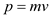Here,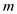is the mass of the object and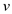is the velocity of the object. So, the linear momentum depends on both the mass and the velocity of the object. The mass of the lineman is more when compared to the mass of the fast runner and the velocity of the fast running person is greater than that of the velocity of the massive lineman. Thus, the velocity of the fast runner will be greater only when the product of the mas and velocity of the fast runner is greater. Therefore, the fast-running running back does not have the more linear momentum than the slow-moving, massive lineman.

The linear momentum acting on an object is,Here,is the mass of the object andis the velocity of the object. Mass ( m ) is 60 kg. Convert the speed of the car from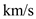to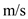(a) The velocity of woman with respect to ground is 25 m/s. Linear momentum is,Substitute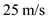forand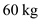for, and solve for the momentum of the woman relative to the ground.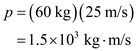Therefore, the linear momentum of the woman relative to the ground is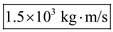. (b) The velocity of woman with respect to car is,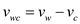Substitute 25 m/s for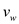and 25 m/s for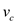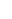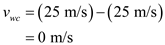Linear momentum is,Substitute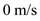for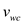andfor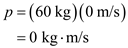Therefore, the linear momentum of the woman relative to the car is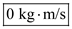.

The linear momentum of an object that undergoing translational motion is defined as the product of mass of the object times the velocity of the object. That is,Here, m is mass of the object and v is the velocity of the object. The SI unit of mass is kg and the SI unit of velocity is m/s. Therefore, the SI unit of linear momentum is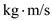. Therefore, the correct option is (b).